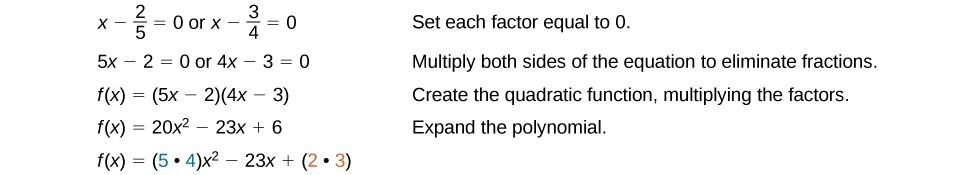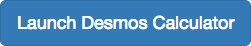## Theorems Used to Analyze Polynomial Functions

### Learning Outcomes

• Evaluate a polynomial using the Remainder Theorem.
• Use the rational zeros theorem to find rational zeros of a polynomial.
• Use the Factor Theorem to solve a polynomial equation.

In the last section, we learned how to divide polynomials. We can now use polynomial division to evaluate polynomials using the Remainder Theorem. If the polynomial is divided by x – k, the remainder may be found quickly by evaluating the polynomial function at k, that is, f(k). Let’s walk through the proof of the theorem.

Recall that the Division Algorithm states that given a polynomial dividend f(x) and a non-zero polynomial divisor d(x) where the degree of d(x) is less than or equal to the degree of f(x), there exist unique polynomials q(x) and r(x) such that

$f\left(x\right)=d\left(x\right)q\left(x\right)+r\left(x\right)$

If the divisor, d(x), is x – k, this takes the form

$f\left(x\right)=\left(x-k\right)q\left(x\right)+r$

Since the divisor x – k is linear, the remainder will be a constant, r. And, if we evaluate this for xk, we have

$\begin{array}{l}f\left(k\right)=\left(k-k\right)q\left(k\right)+r\hfill \\ \text{}f\left(k\right)=0\cdot q\left(k\right)+r\hfill \\ \text{}f\left(k\right)=r\hfill \end{array}$

In other words, f(k) is the remainder obtained by dividing f(x) by x – k.

### A General Note: The Remainder Theorem

If a polynomial $f\left(x\right)$ is divided by x – k, then the remainder is the value $f\left(k\right)$.

### How To: Given a polynomial function $f$, evaluate $f\left(x\right)$ at $x=k$ using the Remainder Theorem

1. Use synthetic division to divide the polynomial by $x-k$.
2. The remainder is the value $f\left(k\right)$.

### Example: Using the Remainder Theorem to Evaluate a Polynomial

Use the Remainder Theorem to evaluate $f\left(x\right)=6{x}^{4}-{x}^{3}-15{x}^{2}+2x - 7$ at $x=2$.

### Try It

Use the Remainder Theorem to evaluate $f\left(x\right)=2{x}^{5}+4{x}^{4}-3{x}^{3}+8{x}^{2}+7$
at $x=-3$.

## Using the Rational Zero Theorem to Find Rational Zeros

Another use for the Remainder Theorem is to test whether a rational number is a zero for a given polynomial, but first we need a pool of rational numbers to test. The Rational Zero Theorem helps us to narrow down the number of possible rational zeros using the ratio of the factors of the constant term and factors of the leading coefficient of the polynomial

Consider a quadratic function with two zeros, $x=\frac{2}{5}$ and $x=\frac{3}{4}$.

These zeros have factors associated with them. Let us set each factor equal to 0 and then construct the original quadratic function.Notice that two of the factors of the constant term, 6, are the two numerators from the original rational roots: 2 and 3. Similarly, two of the factors from the leading coefficient, 20, are the two denominators from the original rational roots: 5 and 4.

We can infer that the numerators of the rational roots will always be factors of the constant term and the denominators will be factors of the leading coefficient. This is the essence of the Rational Zero Theorem; it is a means to give us a pool of possible rational zeros.

### A General Note: The Rational Zero Theorem

The Rational Zero Theorem states that if the polynomial $f\left(x\right)={a}_{n}{x}^{n}+{a}_{n - 1}{x}^{n - 1}+…+{a}_{1}x+{a}_{0}$ has integer coefficients, then every rational zero of $f\left(x\right)$ has the form $\frac{p}{q}$ where p is a factor of the constant term ${a}_{0}$ and q is a factor of the leading coefficient ${a}_{n}$.

When the leading coefficient is 1, the possible rational zeros are the factors of the constant term.

### How To: Given a polynomial function $f\left(x\right)$, use the Rational Zero Theorem to find rational zeros

1. Determine all factors of the constant term and all factors of the leading coefficient.
2. Determine all possible values of $\frac{p}{q}$, where p is a factor of the constant term and q is a factor of the leading coefficient. Be sure to include both positive and negative candidates.
3. Determine which possible zeros are actual zeros by evaluating each case of $f\left(\frac{p}{q}\right)$.

### Example: Listing All Possible Rational Zeros

List all possible rational zeros of $f\left(x\right)=2{x}^{4}-5{x}^{3}+{x}^{2}-4$.

### Example: Using the Rational Zero Theorem to Find Rational Zeros

Use the Rational Zero Theorem to find the rational zeros of $f\left(x\right)=2{x}^{3}+{x}^{2}-4x+1$.

### Try It

Use the Rational Zero Theorem to find the rational zeros of $f\left(x\right)={x}^{3}-3{x}^{2}-6x+8$.

## Using the Factor Theorem to Solve a Polynomial Equation

The Factor Theorem is another theorem that helps us analyze polynomial equations. It tells us how the zeros of a polynomial are related to the factors. Recall that the Division Algorithm tells us $f\left(x\right)=\left(x-k\right)q\left(x\right)+r$.

If k is a zero, then the remainder r is $f\left(k\right)=0$ and $f\left(x\right)=\left(x-k\right)q\left(x\right)+0$ or $f\left(x\right)=\left(x-k\right)q\left(x\right)$.

Notice, written in this form, x – k is a factor of $f\left(x\right)$. We can conclude if is a zero of $f\left(x\right)$, then $x-k$ is a factor of $f\left(x\right)$.

Similarly, if $x-k$ is a factor of $f\left(x\right)$, then the remainder of the Division Algorithm $f\left(x\right)=\left(x-k\right)q\left(x\right)+r$ is 0. This tells us that k is a zero.

This pair of implications is the Factor Theorem. As we will soon see, a polynomial of degree n in the complex number system will have n zeros. We can use the Factor Theorem to completely factor a polynomial into the product of n factors. Once the polynomial has been completely factored, we can easily determine the zeros of the polynomial.

### A General Note: The Factor Theorem

According to the Factor Theorem, k is a zero of $f\left(x\right)$ if and only if $\left(x-k\right)$ is a factor of $f\left(x\right)$.

### How To: Given a factor and a third-degree polynomial, use the Factor Theorem to factor the polynomial

1. Use synthetic division to divide the polynomial by $\left(x-k\right)$.
2. Confirm that the remainder is 0.
3. Write the polynomial as the product of $\left(x-k\right)$ and the quadratic quotient.
4. If possible, factor the quadratic.
5. Write the polynomial as the product of factors.

### Example: Using the Factor Theorem to Solve a Polynomial Equation

Show that $\left(x+2\right)$ is a factor of ${x}^{3}-6{x}^{2}-x+30$. Find the remaining factors. Use the factors to determine the zeros of the polynomial.

### Try It

Use the Factor Theorem to find the zeros of $f\left(x\right)={x}^{3}+4{x}^{2}-4x - 16$ given that $\left(x - 2\right)$ is a factor of the polynomial.Now use an online graphing tool to graph $f(x)$.
On the next line, enter $g(x) = \frac{f(x)}{(x-2)}$.
Where does $g(x)$ cross the x-axis? How do those roots compare to the solution we found using the Factor Theorem?

## Contribute!

Did you have an idea for improving this content? We’d love your input.